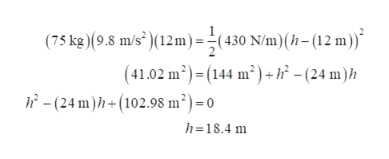# A 75 kg student jumps off a bridge with a 12-m-long bungee cord tied to his feet. The massless bungee cord has a spring constant of 430 N/m.a)    How far below the bridge is the student’s lowest point?b)   How far below the bridge is the student's resting position after the oscillations have fully stopped?

Question
49 views

A 75 kg student jumps off a bridge with a 12-m-long bungee cord tied to his feet. The massless bungee cord has a spring constant of 430 N/m.

a)    How far below the bridge is the student’s lowest point?

b)   How far below the bridge is the student's resting position after the oscillations have fully stopped?

check_circle

Step 1

(a) The gravitational potential energy is converted into the spring potential energy

Step 2

Substitute the values,help_outlineImage Transcriptionclose(75 kg)(9.8 m/s²)(12m)=÷ (430 N/m)(h- (12 m))* (41.02 m²) = (144 m²) +h² - (24 m)h h - (24 m)h + (102.98 m²) = 0 h=18.4 m fullscreen
Step 3

(b) When the oscillation is died of, the gravitational force acting on the jumper is equal t...

### Want to see the full answer?

See Solution

#### Want to see this answer and more?

Solutions are written by subject experts who are available 24/7. Questions are typically answered within 1 hour.*

See Solution
*Response times may vary by subject and question.
Tagged in

### Work,Power and Energy